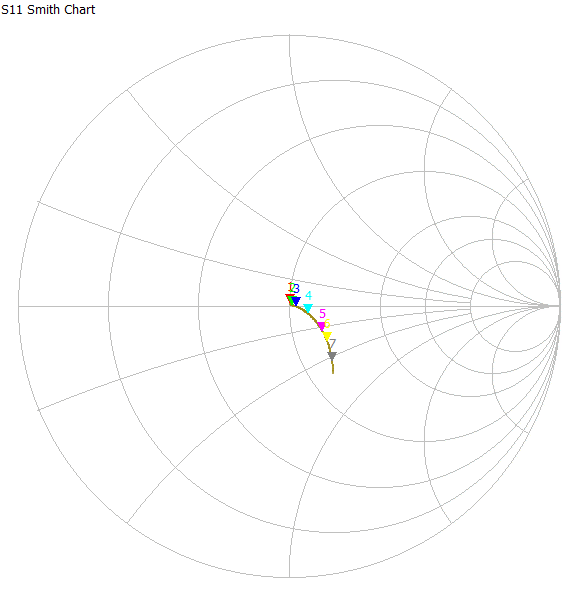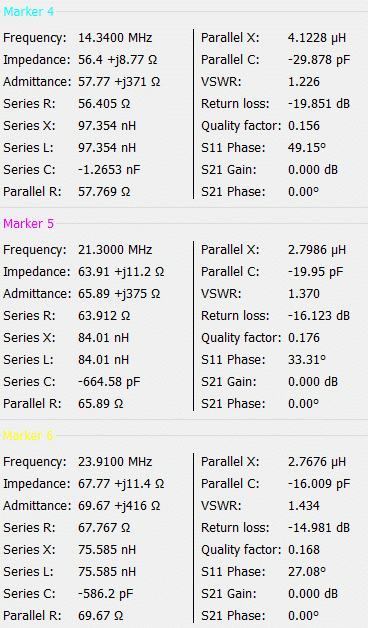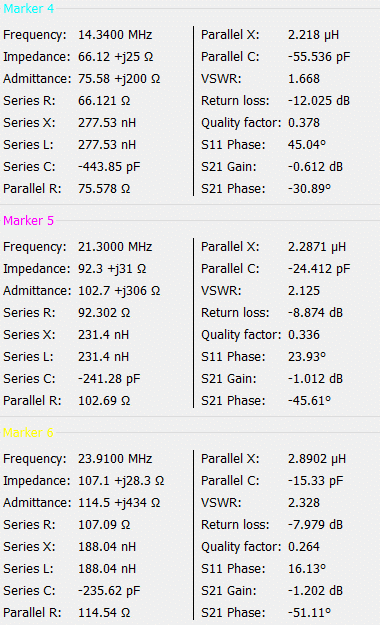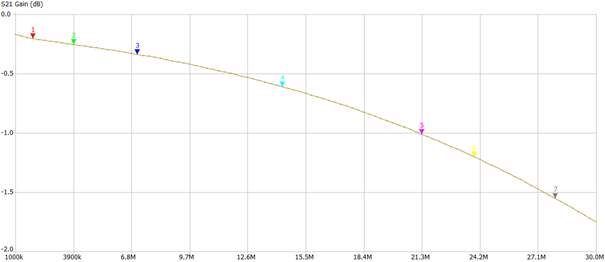Search
• xtgaby

# 50 to 100 ohm transformer for receiving systems.

Updated: Oct 1, 20205 turns input 7 turns output

Used material: 1 core of 73 material Fair-Rite 2873000202Input = 5 turns / Output = 7 turns

5 square = 25 / 7 square = 49

49 / 25 = 2

When Input impedance = 50 Ohms,

Output impedance wil be 2 x 50 Ohm = 100 OhmsOptimization for 7 MHZ: 2 x 39 pF parallel.Optimization for the entire frequency band with 39 pF:Insertion loss for 2 devices:Insertion loss @ 7 MHz = 0.25 / 2 = 0.12 dB